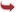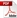International Journal of Scientific and Research Publications

#### IJSRP, Volume 3, Issue 1, January 2013 Edition [ISSN 2250-3153]Fuzzy Logic Gates in Electronic Circuits
G.Nirmala, G.Suvitha
Abstract: In this paper, we can see a brief description to fuzzy logic operation and Logic gates. Here we see how Fuzzy Logic extends and generalizes classical logic with propositional logic. Proposition logic deals with finding truth values of formulas containing atomic propositions, whose truth value is either zero or one, connected by “and” () “or” (), implication () etc...Finally we find truth values of formulas using a t-norm for  and a t-co norm for .
[VIEW FULL PAPER][DOWNLOAD]

Reference this Research Paper (copy & paste below code):

G.Nirmala, G.Suvitha (2018); Fuzzy Logic Gates in Electronic Circuits; Int J Sci Res Publ 3(1) (ISSN: 2250-3153). http://www.ijsrp.org/research-paper-1301.php?rp=P13584